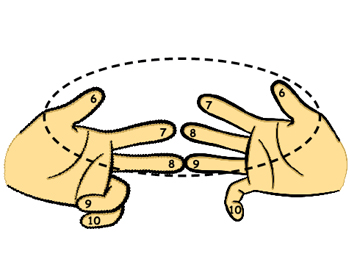Remember that when we multiply 8 and 9 by fingers, we joined finger 8 on the left hand to finger 9 on the right hand.

The two joined fingers and all the fingers above them are called TENS fingers. The fingers below the joined fingers are called ONES fingers.Note

• There are 3 TENS finger on the left hand and 4 TENS fingeron the right hand.

• There are 2 ONES finger on the left hand and 1 ONES finger on the right hand.

• The number of ONES (32) and TENS (4 + 1) fingers on each hand always add up to 5.

Also note how our numbers (8 and 9) relate to the numbers of ONES and TENS fingers.

8 = 10 – (number of ONES finger on left) = 10 – 2 and
9 = 10 – (number of ONES finger on right) = 10 – 1.

Therefore, 8 x 9 = (10 – 2) x (10 – 1)
= 10 x 10 – [10 x (2 + 1)] + (2 x 1)
= 10 x (10 – (2 + 1)) + (2 x 1)
= 10 x [(5 – 2) + (5 – 1)] + (2 x 1)
= 10 x [3 + 4] + (2 x 1)Sum of TENs   Product of ONEs

In general, when we multiply a and b, where a and b are whole numbers from 6 to 10, let

L = 10 – a = number of ONES finger on the left, and
R = 10 – b = number of ONES finger on the right.

So, a x b = (10 – L) x (10 – R)
= 10 x 10 -10 x (L + R) + (L x R)
= 10 x (10 – (L + R)) + L x R
= 10 x [(5 – L) + (5 – R)] + L x RSum of TENs      Product of ONEs

Note that:
5 – L = number of TENS finger on the left and
5 – R = number of ONES finger on the right.
To Top